### Home > CALC > Chapter 4 > Lesson 4.1.2 > Problem4-20

4-20.
1. Differentiate each function below. That is, find its slope function, f ′(x). Homework Help ✎

1. f(x) = 6(x − 2)3

2. f(x) = 2 sin x

3. f(x) = (x + 5)(2x − l)

4.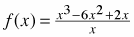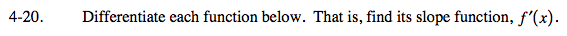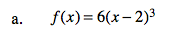You could expand f(x) before you differentiate. Or...

Strategy 1: f '(x) = 18x² − 72x + 72
Strategy 2: f '(x) = 18(x − 2)²

You know that the derivative of y = (x − 2)³ is y ' = 3(x − 2)². What about the vertical stretch of 6? If the f(x) is stretched by a factor of 6, what happens to its slopes/derivative? Are they stretched as well?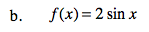What does the 2 do to a sine graph? How will this affect the slope function?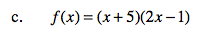Before you differentiate, expand f(x).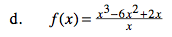Before you differentiate, simplify f(x). But, don't forget that there was once a 0 in the denominator... be sure to restrict the domain of the derivative.